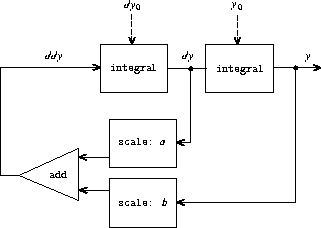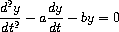# Solve-2nd procedureFigure 3.35: Signal-flow diagram for the solution to a second-order linear differential equation.

Consider the problem of designing a signal-processing system to study the homogeneous second-order linear differential equationThe output stream, modeling `y` , is generated by a network that contains a loop. This is because the value of `d²y/dt²` depends upon the values of `y` and `dy/dt` and both of these are determined by integrating `d²y/dt²` . The diagram we would like to encode is shown in figure 3.35. Write a procedure `solve-2nd` that takes as arguments the constants `a` , `b` , and `dt` and the initial values `y₀` and `dy₀` for `y` and `dy/dt` and generates the stream of successive values of `y` .